Related Articles

• Last Updated : 23 Jul, 2019

In Windows Forms, RadioButton control is used to select a single option among the group of the options. For example, select your gender from the given list, so you will choose only one option among three options like Male or Female or Transgender. In C#, RadioButton is a class and it is defined under System.Windows.Forms namespace. In RadioButton, you are allowed to display text, image, or both and when you select one radio button in a group other radio buttons automatically clear. You can create RadioButton in two different ways:

1. Design-Time: It is the easiest way to create a RadioButton control as shown in the following steps:

• Step 1: Create a windows form as shown in the below image:
Visual Studio -> File -> New -> Project -> WindowsFormApp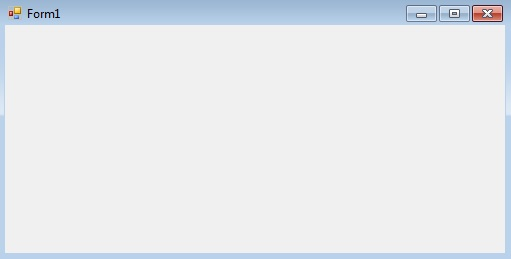• Step 2: Drag the RadioButton control from the ToolBox and drop it on the windows form. You are allowed to place a RadioButton control anywhere on the windows form according to your need.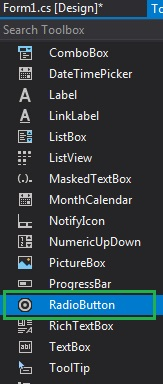• Step 3: After drag and drop you will go to the properties of the RadioButton control to modify RadioButton control according to your requirment.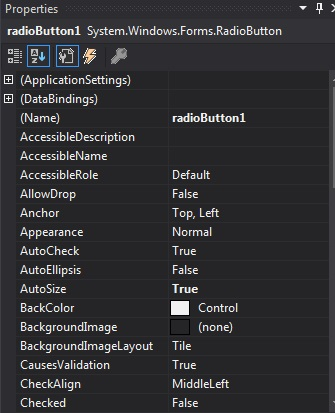Output: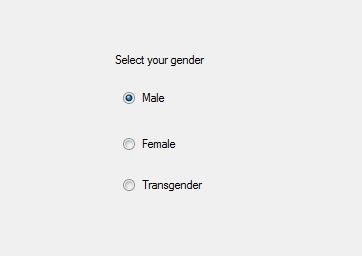2. Run-Time: It is a little bit trickier than the above method. In this method, you can create a RadioButton programmatically with the help of the RadioButton class. The following steps show how to create a RadioButton dynamically:

• Step 1: Create a radio button using the RadioButton() constructor is provided by the RadioButton class.
```// Creating radio button
```
• Step 2: After creating RadioButton, set the properties of the RadioButton provided by the RadioButton class.
```// Set the AutoSize property
r1.AutoSize = true;

r1.Text = "Intern";

// Set the location of the RadioButton
r1.Location = new Point(286, 40);

// Set Font property
r1.Font = new Font("Berlin Sans FB", 12);
```
```// Add this radio button to the form
```

Example:

 `using` `System;``using` `System.Collections.Generic;``using` `System.ComponentModel;``using` `System.Data;``using` `System.Drawing;``using` `System.Linq;``using` `System.Text;``using` `System.Threading.Tasks;``using` `System.Windows.Forms;`` ` `namespace` `WindowsFormsApp24 {`` ` `public` `partial` `class` `Form1 : Form {`` ` `    ``public` `Form1()``    ``{``        ``InitializeComponent();``    ``}`` ` `    ``private` `void` `RadioButton2_CheckedChanged(``object` `sender,``                                               ``EventArgs e)``    ``{``    ``}`` ` `    ``private` `void` `Form1_Load(``object` `sender, EventArgs e)``    ``{``        ``// Creating and setting label``        ``Label l = ``new` `Label();``        ``l.AutoSize = ``true``;``        ``l.Location = ``new` `Point(176, 40);``        ``l.Text = ``"Select Post:"``;``        ``l.Font = ``new` `Font(``"Berlin Sans FB"``, 12);`` ` `        ``// Adding this label to the form``        ``this``.Controls.Add(l);`` ` `        ``// Creating and setting the ``        ``// properties of the RadioButton``        ``RadioButton r1 = ``new` `RadioButton();``        ``r1.AutoSize = ``true``;``        ``r1.Text = ``"Intern"``;``        ``r1.Location = ``new` `Point(286, 40);``        ``r1.Font = ``new` `Font(``"Berlin Sans FB"``, 12);`` ` `        ``// Adding this label to the form``        ``this``.Controls.Add(r1);`` ` `        ``// Creating and setting the ``        ``// properties of the RadioButton``        ``RadioButton r2 = ``new` `RadioButton();``        ``r2.AutoSize = ``true``;``        ``r2.Text = ``"Team Leader"``;``        ``r2.Location = ``new` `Point(356, 40);``        ``r2.Font = ``new` `Font(``"Berlin Sans FB"``, 12);``        ``// Adding this label to the form``        ``this``.Controls.Add(r2);`` ` `        ``// Creating and setting the ``        ``// properties of the RadioButton``        ``RadioButton r3 = ``new` `RadioButton();``        ``r3.AutoSize = ``true``;``        ``r3.Text = ``"Software Engineer"``;``        ``r3.Location = ``new` `Point(480, 40);``        ``r3.Font = ``new` `Font(``"Berlin Sans FB"``, 12);`` ` `        ``// Adding this label to the form``        ``this``.Controls.Add(r3);``    ``}``}``}`

Output: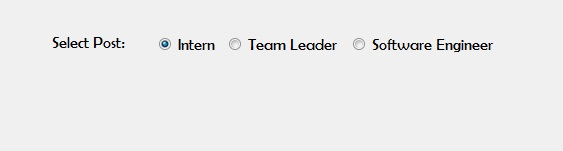#### Important Events

My Personal Notes arrow_drop_up